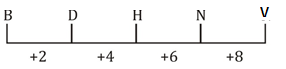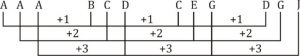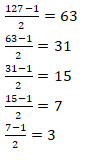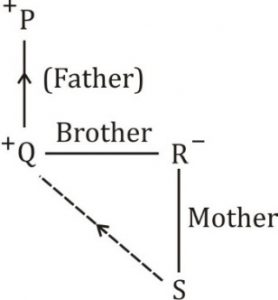# Reasoning for SSC CHSL,CGL 18th January 2020 for Dictionary and odd one out

Direction (1-2): In the following question, select the odd word from the given alternatives.

Q1.
(a) 8
(b) 27
(c) 49
(d) 216

Q2.
(a) 123 – 321
(b) 456 – 654
(c) 789 – 978
(d) 678 – 876

Direction (3-4): Arrange the given words in the sequence in which they occur in the dictionary.

Q3.1. Clone      2. Climate
3. Clutter    4. Create
5. Clapped
(a) 52143
(b) 51234
(c) 52134
(d) 53124

Q4.1. Lasted     2. Loop
3. Lake            4. Litter
5. Listed
(a) 31542
(b) 31254
(c) 31245
(d) 31452

Direction (5-6): A series is given with one term missing. Select the correct alternative from the given ones that will complete the series.

Q5. B, D, H, N, ?
(a) T
(b) U
(c) V
(d) W

Q6. AAA, BCD, CEG, ?
(a) DGI
(b) DFI
(c) DGJ
(d) DJG

Direction (7-8): In the following question, select the missing number from the given series.

Q7. 127, 63, 31, 15, 7, ?
(a) 2
(b) 3
(c) 4
(d) 5

Q8. 2, 22, 198, 1386, ?
(a) 2770
(b) 3990
(c) 6930
(d) 9702

Q9. A is taller than B. C is taller than D, but shorter than E. B is shorter than D and D is taller than A. Who is the tallest?
(a) E
(b) C
(c) B
(d) D

Q10. If ‘A + B’ means ‘A is father of B’, ‘A – B’ means ‘A is mother of B’, ‘A * B’ means ‘A is brother of B’ and ‘A % B’ means ‘A is sister of B’, then how is Q related to
S in ‘P + Q * R – S’?
(a) Husband
(b) Uncle
(c) Brother
(d) Father

#### Solutions:

S1. Ans. (c);
Sol. All are perfect cubes except 49.

S2. Ans. (c);
Sol. Numbers are written is reverse sequence.

S3. Ans. (c);
Sol. 5. Clapped
2. Climate
1. Clone
3. Clutter
4. Create

S4. Ans. (a);
Sol. 3. Lake
1. Lasted
5. Listed
4. Litter
2. Loop

S5. Ans. (c);
Sol.S6. Ans. (c);
Sol.S7. Ans. (b);
Sol.S8. Ans (c);
Sol. 2×11=22
22×9=198
198×7=1386
1386×5=6930

S9. Ans. (a);
Sol. E > C > D > A > B
E is the tallest.

S10. Ans. (b);
Sol.Q is the uncle of S.

##### SSC CGL | SSC CHSL 2020 | Free Marathon Class English, Science, Maths, Reasoning

You may also like to read: